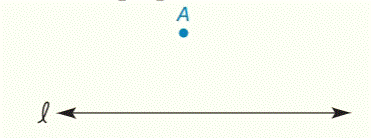Chapter 2.CT, Problem 6CT### Elementary Geometry for College St...

6th Edition
Daniel C. Alexander + 1 other
ISBN: 9781285195698

#### Solutions

Chapter
Section### Elementary Geometry for College St...

6th Edition
Daniel C. Alexander + 1 other
ISBN: 9781285195698
Textbook Problem
1 views

# Through the point A, construct the line that is perpendicular to line l .To determine

To draw:

A line that is perpendicular to line l through the point A.

Explanation

Given:

The following figure shows the given diagram.

Figure (1)

Approach:

Consider the point A as center and draw the circle that intersects the line l as shown below.

Figure (2)

Consider the points D and E and draw the circle having radius equal to the DA and get intersecting points of the two circles as shown below

### Still sussing out bartleby?

Check out a sample textbook solution.

See a sample solution

#### The Solution to Your Study Problems

Bartleby provides explanations to thousands of textbook problems written by our experts, many with advanced degrees!

Get Started

#### In Problems 1-16, use integration by parts to evaluate the integral. 5.

Mathematical Applications for the Management, Life, and Social Sciences

#### Evaluate the integral. 4. sin3xcosxdx

Single Variable Calculus: Early Transcendentals

#### Evaluate dx using the Fundamental Theorem of Calculus. a) 7 b) 9 c) d) 18

Study Guide for Stewart's Single Variable Calculus: Early Transcendentals, 8th